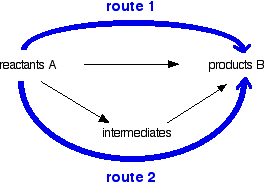# Hess's Law and Simple Enthalpy Calculations

$$\newcommand{\vecs}{\overset { \rightharpoonup} {\mathbf{#1}} }$$ $$\newcommand{\vecd}{\overset{-\!-\!\rightharpoonup}{\vphantom{a}\smash {#1}}}$$$$\newcommand{\id}{\mathrm{id}}$$ $$\newcommand{\Span}{\mathrm{span}}$$ $$\newcommand{\kernel}{\mathrm{null}\,}$$ $$\newcommand{\range}{\mathrm{range}\,}$$ $$\newcommand{\RealPart}{\mathrm{Re}}$$ $$\newcommand{\ImaginaryPart}{\mathrm{Im}}$$ $$\newcommand{\Argument}{\mathrm{Arg}}$$ $$\newcommand{\norm}{\| #1 \|}$$ $$\newcommand{\inner}{\langle #1, #2 \rangle}$$ $$\newcommand{\Span}{\mathrm{span}}$$ $$\newcommand{\id}{\mathrm{id}}$$ $$\newcommand{\Span}{\mathrm{span}}$$ $$\newcommand{\kernel}{\mathrm{null}\,}$$ $$\newcommand{\range}{\mathrm{range}\,}$$ $$\newcommand{\RealPart}{\mathrm{Re}}$$ $$\newcommand{\ImaginaryPart}{\mathrm{Im}}$$ $$\newcommand{\Argument}{\mathrm{Arg}}$$ $$\newcommand{\norm}{\| #1 \|}$$ $$\newcommand{\inner}{\langle #1, #2 \rangle}$$ $$\newcommand{\Span}{\mathrm{span}}$$$$\newcommand{\AA}{\unicode[.8,0]{x212B}}$$

Hess's Law is used to do some simple enthalpy change calculations involving enthalpy changes of reaction, formation and combustion. The enthalpy change accompanying a chemical change is independent of the route by which the chemical change occurs.

Hess's Law is the most important law in this part of chemistry and most calculations follow from it. Hess's Law is saying that if you convert reactants A into products B, the overall enthalpy change will be exactly the same whether you do it in one step or two steps or however many steps. If you look at the change on an enthalpy diagram, that is actually fairly obvious.This shows the enthalpy changes for an exothermic reaction using two different ways of getting from reactants A to products B. In one case, you do a direct conversion; in the other, you use a two-step process involving some intermediates.

In either case, the overall enthalpy change must be the same, because it is governed by the relative positions of the reactants and products on the enthalpy diagram. If you go via the intermediates, you do have to put in some extra heat energy to start with, but you get it back again in the second stage of the reaction sequence. However many stages the reaction is done in, ultimately the overall enthalpy change will be the same, because the positions of the reactants and products on an enthalpy diagram will always be the same.

You can do calculations by setting them out as enthalpy diagrams as above, but there is a much simpler way of doing it which needs virtually no thought. You could set out the above diagram as:Hess's Law says that the overall enthalpy change in these two routes will be the same. That means that if you already know two of the values of enthalpy change for the three separate reactions shown on this diagram (the three black arrows), you can easily calculate the third - as you will see below. The big advantage of doing it this way is that you don't have to worry about the relative positions of everything on an enthalpy diagram. It is completely irrelevant whether a particular enthalpy change is positive or negative.

Although most calculations you will come across will fit into a triangular diagram like the above, you may also come across other slightly more complex cases needing more steps. You need to take care in choosing your two routes. The pattern will not always look like the one above.

##### Jim Clark (Chemguide.co.uk)

This page titled Hess's Law and Simple Enthalpy Calculations is shared under a CC BY-NC 4.0 license and was authored, remixed, and/or curated by Jim Clark.